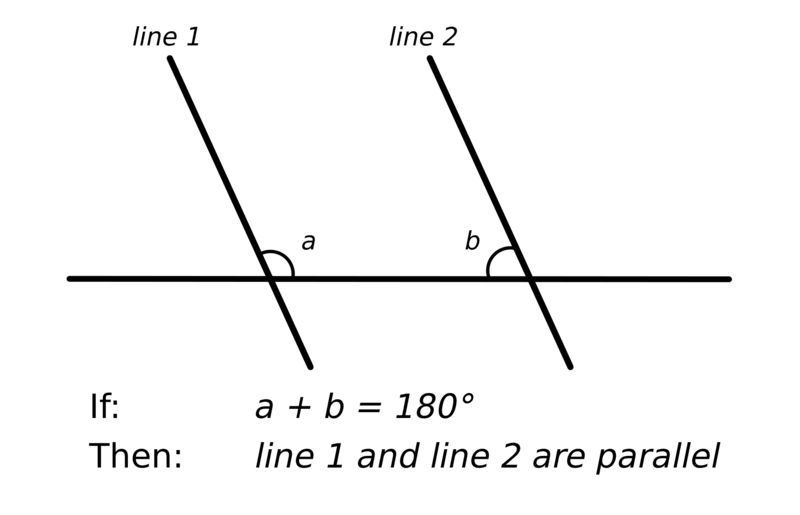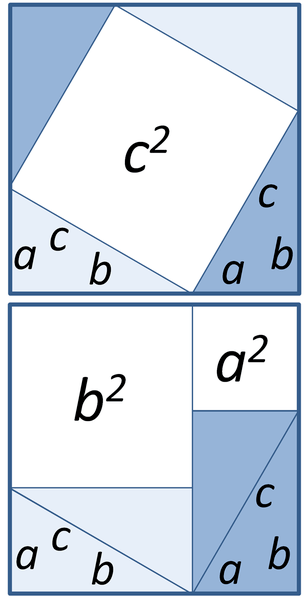# What is the Difference Between Postulates and Theorems

The main difference between postulates and theorems is that postulates are assumed to be true without any proof while theorems can be and must be proven to be true.

Theorems and postulates are two concepts you find in geometry. In fact, these are statements of geometrical truth. Postulates are the ideas that are thought to be obviously true that they do not require proof. Theorems are mathematical statements that we can/must prove to be true.

### Key Areas Covered

1. What are Postulates
– Definition, Characteristics
2. What are Theorems
– Definition, Characteristics
3. Relationship Between Postulates and Theorems
– Outline of Common Features
4. Difference Between Postulates and Theorems
– Comparison of Key Differences

### Key Terms

Postulates, Theorems## What are Postulates

Postulates are the mathematical statements we assume to be true without any proof. They are ideas we consider to be so obviously true that they do not require proof. For instance, the statement that a line contains at least two points is a postulate. This is so obvious and generally accepted that we don’t usually require any proof to accept it as true. We create theorems and lemmas on the basis of theorems. In fact, it possible to create a theorem from one or more postulate.Figure 1: Euclid’s Parallel Postulate

### Basic Characteristics of Postulates

• A postulate must be easy to understand – for example; it should not have a lot of words that are difficult to understand.
• They should be consistent when combined with other postulates.
• We should be able to use them independently.

However, it’s also important to note that some postulates are not always correct. A postulate may prove to be incorrect after a new discovery. For example, Einstein’s postulate that the universe is homogenous is no longer accepted as correct.

## What are Theorems

A theorem is a mathematical statement we can prove as true. We can prove them by using logical reasoning or by using other theorems that have been already proven true. In fact, A theorem that has to be proved in order to prove another theorem is called a lemma. Postulates are the basis on which we build both lemmas and theorems. Four colour theorem, Pythagorean theorem, and Fermat’s Last Theorem are some examples of theorems.Figure 02: Pythagorean Theorem

### Pythagorean Theorem

According to Pythagorean Theorem, when a triangle has a right angle (90°), and each of the three sides of the triangle makes up squares, the biggest square has the same area as the other two squares put together. In other words, the square of the hypotenuse of a right-angled triangle is equal to the sum of the squares of the other two sides. We can indicate this using the equation a2 + b2 = c2.

## Relationship Between Postulates and Theorems

• Theorems and postulates are statements of geometrical truth.
• We create theorems based on postulates.

## Difference Between Postulates and Theorems

### Definition

Postulates are the mathematical statements we assume to be true without any proof while theorems are mathematical statements we can or must prove as true.

### Proof

Postulates are assumed to be true without any proof, while theorems can be proven as true.

### Need for Proof

Furthermore, we don’t need to prove postulates because they state the obvious, but theorems are not so obvious and can be proven by logical reasoning or using lemmas.

### Conclusion

Postulates are the mathematical statements we assume to be true without any proof while theorems are mathematical statements we can or must prove as true. Hence, the main difference between postulates and theorems is their proof.

##### Reference:

1. “Postulates and Theorems.” CliffNotes, Available here.

##### Image Courtesy:

1. “Parallel Postulate” By Alecmconroy at the English language Wikipedia (CC BY-SA 3.0) via Commons Wikimedia
2. “Pythagorean Proof (3)” By Brews ohare – Own work (CC BY-SA 3.0) via Commons Wikimedia